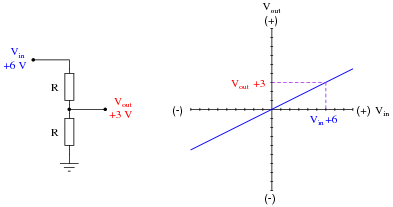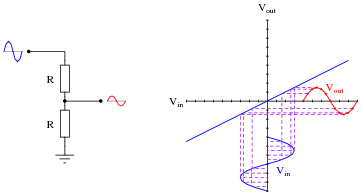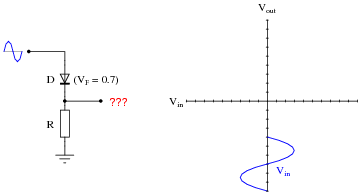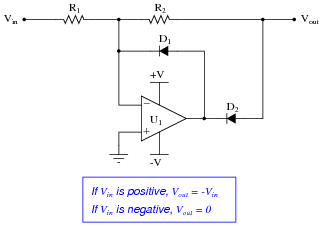# Precise Diode Circuits

• #### Question 1

 Don’t just sit there! Build something!!

Learning to mathematically analyze circuits requires much study and practice. Typically, students practice by working through lots of sample problems and checking their answers against those provided by the textbook or the instructor. While this is good, there is a much better way.

You will learn much more by actually building and analyzing real circuits, letting your test equipment provide the “answers” instead of a book or another person. For successful circuit-building exercises, follow these steps:

1. Carefully measure and record all component values prior to circuit construction.
2. Draw the schematic diagram for the circuit to be analyzed.
3. Carefully build this circuit on a breadboard or other convenient medium.
4. Check the accuracy of the circuit’s construction, following each wire to each connection point, and verifying these elements one-by-one on the diagram.
5. Mathematically analyze the circuit, solving for all voltage and current values.
6. Carefully measure all voltages and currents, to verify the accuracy of your analysis.
7. If there are any substantial errors (greater than a few percent), carefully check your circuit’s construction against the diagram, then carefully re-calculate the values and re-measure.

Avoid using the model 741 op-amp, unless you want to challenge your circuit design skills. There are more versatile op-amp models commonly available for the beginner. I recommend the LM324 for DC and low-frequency AC circuits, and the TL082 for AC projects involving audio or higher frequencies.

As usual, avoid very high and very low resistor values, to avoid measurement errors caused by meter “loading”. I recommend resistor values between 1 kΩ and 100 kΩ.

One way you can save time and reduce the possibility of error is to begin with a very simple circuit and incrementally add components to increase its complexity after each analysis, rather than building a whole new circuit for each practice problem. Another time-saving technique is to re-use the same components in a variety of different circuit configurations. This way, you won’t have to measure any component’s value more than once.

• #### Question 2

A common type of graph used to describe the operation of an electronic component or sub-circuit is the transfer characteristic, showing the relationship between input signal and output signal. For example, the transfer characteristic for a simple resistive voltage divider circuit is a straight line:Once a transfer characteristic has been plotted, it may be used to predict the output signal of a circuit given any particular input signal. In this case, the transfer characteristic plot for the 2:1 voltage divider circuit tells us that the circuit will output 3 volts for an input of 6 volts:We may use the same transfer characteristic to plot the output of the voltage divider given an AC waveform input:While this example (a voltage divider with a 2:1 ratio) is rather trivial, it shows how transfer characteristics may be used to predict the output signal of a network given a certain input signal condition. Where transfer characteristic graphs are more practical is in predicting the behavior of nonlinear circuits. For example, the transfer characteristic for an ideal half-wave rectifier circuit looks like this:Sketch the transfer characteristic for a realistic diode (silicon, with 0.7 volts forward drop), and use this characteristic to plot the half-wave rectified output waveform given a sinusoidal input:• #### Question 3

Determine the output voltage of this circuit, assuming a silicon diode (0.7 volts typical forward drop):Now, determine the output voltage of the same circuit with a Schottky diode (0.4 volts typical forward drop) instead of a silicon PN junction diode:Now, determine the output voltage of the same circuit with a light-emitting diode (1.7 volts typical forward drop):Comment on these three circuits’ output voltages: what does this indicate about the effect of the diode’s voltage drop on the opamp output?

• #### Question 4

Determine the output voltage of this circuit for two different input voltage values: 5 volts, and -5 volts, assuming the use of ordinary silicon rectifying diodes:Based on this data (and any other input conditions you wish to test this circuit under), describe what the function of this circuit is.

• #### Question 5

This opamp circuit is called a precision rectifier. Analyze its output voltage as the input voltage smoothly increases from -5 volts to 5 volts, and explain why the circuit is worthy of its name:Assume that both diodes in this circuit are silicon switching diodes, with a nominal forward voltage drop of 0.7 volts.

• #### Question 6

Explain why the following opamp circuit cannot be used as a rectifier in an AC-DC power supply circuit:• #### Question 7

Predict how the operation of this precision rectifier circuit will be affected as a result of the following faults. Consider each fault independently (i.e. one at a time, no multiple faults):Resistor R1 fails open:
Resistor R2 fails open:
Diode D1 fails open:
Diode D2 fails open:

For each of these conditions, explain why the resulting effects will occur.

• #### Question 8

The following circuit is sometimes referred to as a polarity separator. Invent some test conditions you would use to “prove” the operation of the circuit, then analyze the circuit under those imagined conditions and see what the results are:Explain what each output does in this “polarity separator” circuit for any given input voltage.

• #### Question 9

Determine the output voltage of this circuit for two different input voltage values: 4 volts, and -4 volts. Determine the voltage at each and every node with respect to ground as part of your analysis:Based on this data (and any other input conditions you wish to test this circuit under), describe what the function of this circuit is.

• #### Question 10

Explain how you could reverse the output polarity of this precision rectifier circuit:• #### Question 11

One problem with PMMC (permanent magnet moving coil) meter movements is trying to get them to register AC instead of DC. Since these meter movements are polarity-sensitive, their needles merely vibrate back and forth in a useless fashion when powered by alternating current:The same problem haunts other measurement devices and circuits designed to work with DC, including most modern analog-to-digital conversion circuits used in digital meters. Somehow, we must be able to rectify the measured AC quantity into DC for these measurement circuits to properly function.

A seemingly obvious solution is to use a bridge rectifier made of four diodes to perform the rectification:The problem here is the forward voltage drop of the rectifying diodes. If we are measuring large voltages, this voltage loss may be negligible. However, if we are measuring small AC voltages, the drop may be unacceptable.

Explain how a precision full-wave rectifier circuit built with an opamp may adequately address this situation.

• #### Question 12

Suppose that diode D1 in this precision rectifier circuit fails open. What effect will this have on the output voltage?Hint: if it helps, draw a table of figures relating Vin with Vout, and base your answer on the tabulated results.

• #### Question 13

Determine the output voltage of this circuit for the following input voltage conditions:

V1 = 2 volts
V3 = −1.5 volts
V1 = 2.2 voltsHint: if you find this circuit too complex to analyze all at once, think of a way to simplify it so that you may analyze it one “piece” at a time.

• #### Question 14

This circuit is referred to as a peak follower-and-hold, taking the last greatest positive input voltage and “holding” that value at the output until a greater positive input voltage comes along:Give a brief explanation of how this circuit works, as well as the purpose and function of the “reset” switch. Also, explain why a FET input opamp is required for the last stage of amplification.